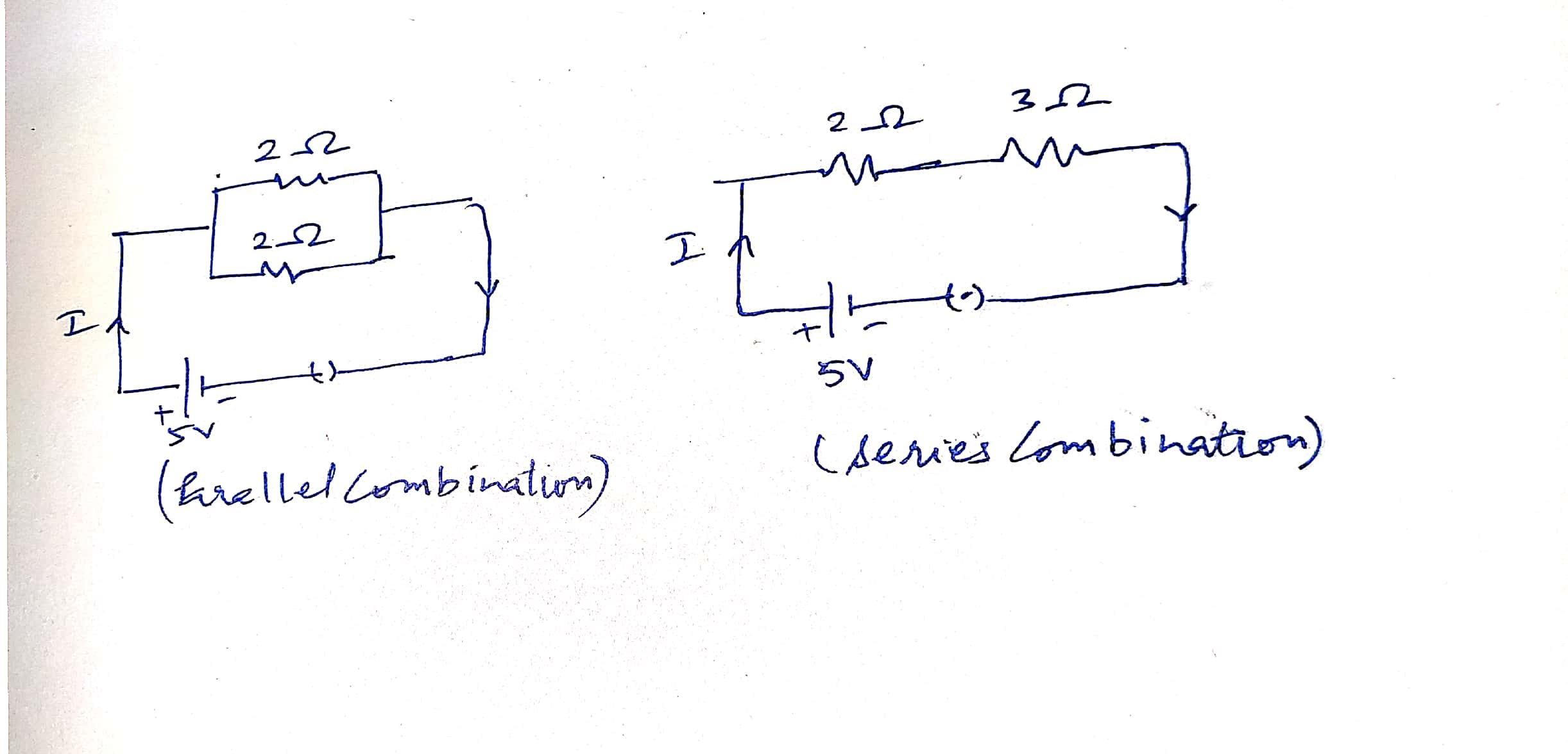Filter By

## All Questions

#### The octahedral complex  exists in two isomeric forms  and  . Isomer  reacts with to give a while precipitate,but does not react with  . Isomer  gives white precipitate with  but does not react with .Isomers and areOption: 1 ionization isomersOption: 2 linkage isomersOption: 3 co-ordination isomersOption: 4 solvate isomers

Ionization isomers have the same central metal ion; only the ligands exchange place with anion or neutral ion outside of the co-ordination complex. The sole difference between the two isomers is which ligands are attached to the central metal.

The X isomer of is and the Y isomer is

Their reactions are as follows:

The correct answer is Option A- Ionization isomers.

#### In a one component second order reaction,if the concentration of the reactant is reduced to half , the rateOption: 1 increases two timesOption: 2 increases four timesOption: 3 decreases to one halfOption: 4 decreases to one fourth

As we have learnt,

For a second order Reaction

So when the [Reactant] is halved, rate will become one-fourths

Hence, the correct answer is Option (4)

## Crack CUET with india's "Best Teachers"

• HD Video Lectures
• Unlimited Mock Tests
• Faculty Support#### When cell has stalled DNA replication fork, which checkpoint should be predominantly activated?Option: 1 G1/S Option: 2 G2/M   Option: 3 M Option: 4 Both G2 M and M

G2/M should be activated as the cell has stalled DNA replication fork.

#### Design an activity to show that chlorophyll is essential for photosynthesis.

Explain two neutralisation reaction relaxed to daily life situations

## JEE Main high-scoring chapters and topics

Study 40% syllabus and score up to 100% marks in JEE

#### (a) Two lamps rated and are connected in parallel to  supply. Calculate the total current through the circuit. (b) Two resistors X and Y of resistances  and respectively are first joined in parallel and then in series. In each case the voltage supplied is . (i) Draw circuit diagrams to show the combination of resistors in each case. (ii) Calculate the voltage across the resistor in the series combination of resistors.

(a)   I1 = P1/V

I1 = 100 W/ 220 V = 10/  22 A

I2 = P2 / V = 10/ 220 = 1/ 22 A

I = I1 + I= = 0.5 A

(b)

(i) Circuit diagrams to show the combination of resistors in each case-(ii) Net R=R1+R2=2+3=5 ?

I = V/ Rnet = 5 / 5 = 1A

∴ Voltage across 3? resistor -

∴ V = 1 × 3 = 3V

#### Give reasons : (a) Ventricles have thicker muscular walls than atria. (b) Transport system in plants is slow. (c) Circulation of blood in aquatic vertebrates differs from that in terrestrial vertebrates. (d) During the daytime, water and minerals travel faster through xylem as compared to the night. (e) Veins have valves whereas arteries do not.

a) because ventricles have to pump blood out of the heart.

b) because plants are not motile and have a large proportion of dead cells in many tissues and due to which their energy needs are low and have a slow transport system.

c) because the terrestrial vertebrates take oxygen from the air through their lungs and the aquatic invertebrates take oxygen from the water through their gills.

d) because the rate of transpiration is higher during the daytime as compared to night.

e) because arteries don't require valves, as the pressure from the heart is so strong that blood is only able to flow in one direction.

## NEET 2024 Most scoring concepts

Just Study 32% of the NEET syllabus and Score up to 100% marks

#### In the question two statements are given one labelled Assertion (A) and the other labelled Reason (R). Select the correct answer to these questions from the codes (i), (ii), (iii) and (iv) as given below. Assertion (A) : At high temperatures, metal wires have a greater chance of short circuiting. Reason (R) : Both resistance and resistivity of a material vary with temperature.   Option: 1 Both (A) and (R) are true and (R) is correct explanation of the assertion (A). Option: 2 Both (A) and (R) are true, but (R) is not the correct explanation of the assertion (A). Option: 3 (A) is true, but (R) is false. Option: 4 (A) is false, but (R) is true.

Both (A) and (R) are true, but (R) is not the correct explanation of the assertion (A).

#### In the question two statements are given one labelled Assertion (A) and the other labelled Reason (R). Select the correct answer to these questions from the codes (i), (ii), (iii) and (iv) as given below. Assertion (A) : Carbon has a strong tendency to either lose or gain electrons to attain noble gas configuration. Reason (R) : Carbon has four electrons in its outermost shell and has the tendency to share electrons with carbon or other                       elements. Option: 1 Both (A) and (R) are true and (R) is correct explanation of the assertion (A). Option: 2 Both (A) and (R) are true, but (R) is not the correct explanation of the assertion (A). Option: 3 (A) is true, but (R) is false. Option: 4 (A) is false, but (R) is true.

(A) is false, but (R) is true.

## Crack CUET with india's "Best Teachers"

• HD Video Lectures
• Unlimited Mock Tests
• Faculty Support#### An elementis forming acidic oxide. Its most probable position in the modern periodic table is Option: 1 Groupand PeriodOption: 2 Groupand PeriodOption: 3 Groupand PeriodOption: 4 Groupand PeriodGroupand Period#### An elementwith atomic numberforms a compound with elementwith atomic number. The formula of the compound formed is Option: 1Option: 2Option: 3Option: 4The formula of the compound formed is